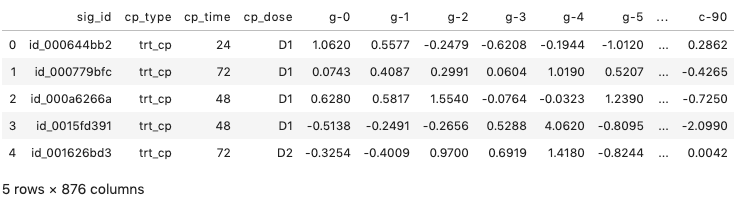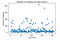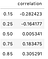# Classifying Mechanism of Action

This post is a reflection on my Mechanism of Action Classification project. It describes how I made decisions on exploratory analysis, cleaning and modeling. My code is available on Github. If you want to discuss anything or find an error, please email me at Lvqingchuan@gmail.com :)

## Brief Background

This project predicts 206 targets of the Mechanism of Action (MoA) response(s) of different samples, given 875 features such as gene expression data and cell viability data. Features g- signify gene expression data, and c- signify cell viability data. Control perturbations (cp_type = ctrl_vehicle) have no MoAs; cp_time and cp_dose indicate treatment duration (24, 48, 72 hours) and dose (high or low). The training data includes 2,3814 unique samples (unique by “sig_id”). The graphs below give you a glance at how training features look:Features in the Training Data

## Exploring Data

This biological research dataset doesn’t have any missing values. All the target labels are binary. Each target has at least one sample classified into it. About 1/10 of 20K unique samples are classified into multiple labels.

The above graph shows the counts of samples by the number of classes they fall in. Most samples (12k) fall in only one class; 9k samples don’t have a class, out of which 1.8k samples with control_vehicle cp type don’t have a class by definition; about 2k samples have multiple classes and most of them have two. Later I dropped 1.8k samples, as they don’t need to go through an algorithm — their cp_type values are control vehicle and therefore are doomed to have no class. As categorical features will be transformed to numerical features later and all variables will be normalized in the PCA step, I don’t have to worry much about the change of their distribution by dropping 1.8k samples here. It’s a relatively small amount of samples being dropped anyway.Number of samples classified into each label

Among labels that have at least one sample classified into, nfkb_inhibitor and proteasome_inhibitor are the most popular labels, with 832 and 726 samples each (still fairly small compared to the total number of 20k samples). Indeed, samples quite often fall in these two classes at the same time: 91% of samples in nfkb_inhibitor are also in the proteasome_inhibitor class. However, they don’t share samples with other labels often. Among 14 labels that they share samples with, the largest number of shared samples is only twelve. This tells me classes are not balanced, and leads to the use of class_weight parameter in the training of Random Forest later.

For algorithms that don’t have a built-in resampling method, I created a new sampling method for this multi-label problem, because academically there’s no mature or universal resampling function for multi-label problems yet. The idea is to group labels according to how many samples they have, then assign a weighted numerical value accordingly. Then, I matched this categorical variable with samples: when a sample was classified into nfkb_inhibitor or proteasome_inhibitor classes, its assigned value is 3; else if a sample was classified into a label with below 600 and above 200 samples, its assigned value is 2; else, its assigned value is 1. This step transferred a multi-label problem to a multi-class problem. I used RandomOverSampler() to upsample minority classes, and obtained a more balanced dataset.

Labels don’t have a strong connection with each other: only 8 pairs out of 206 labels have over 40% correlation. It’s the opposite for features: 30% of pairs of 875 features have over 30% correlation. This leads me to use PCA to reduce dimensionality and correlations of features later— to save runtime and reduce overfitting in the modeling phase.Quantiles of Feature Correlation

The outlier detection of this dataset takes a long runtime, as there’s over eight hundred features. Here, I define an outlier to be less than Q1-IQR or greater than Q3+IQR, where Q# stands for quantile # and IQR is the interquantile range (Q3-Q1). Following this definition, each feature has at least 950 and at most 5k outliers. By looking into the contexts of mechanism of action, I figure these “outliers” are important signals. As a cell indicator or gene indicator signals extreme values, it’s more likely this sample corresponds to a certain type of labels. Therefore, I decide to keep these extreme values.

Only three features are categorical. Actually two if you don’t count the sample id column. By deleting all the rows with cp_type equals to control_vehicle, the cp_type column can only be trt_cp and thus loses prediction power. Therefore, I deleted the cp_type feature, and transferred the only categorical feature left, cp_dose, with Label Encoder for Random Forest. Note that cp_time is numerical categorical features and can equal to 24, 48 or 72 hours. I don’t think it’s necessary to encode this variable by 0, 1 and 2.

Training Models

When training models, I built PCA pipelines within cross-validation framework to search for the optimal variability of PCA and other hyperparameters. PCA is very convenient here for three reasons: algorithms that use distance metrics, such as KNN and MLKNN, are sensitive to the scales of data; neural network, such as MLP, will run much faster with scaled data and not saturate too fast; PCA helps reduce correlation among features — saves algorithms extra work to understand high correlated features and reduces the chances of overfitting.

I trained four models: Random Forest, KNN, MLKNN and MLP. SVM doesn’t fit in here, because either one-vs-one or one-vs-rest predicts one single value for a sample. A get-around could be building a SVM for each of 206 labels, but that’s too time-consuming. For each of model, I used gradient search to tune hyperparameters with three cross-validation folds. A few points of models that might worth mentioning:

• class_weight = “balanced” when training Random Forest. This mode automatically adjusts weights inversely proportional to class frequencies in the input data as n_samples/(n_classes * np.bincount(y)). As mentioned above, nfkb_inhibitor and proteasome_inhibitor are the most popular labels and we have an imbalanced set of labels.
• The smallest number of neighborhoods is five when training KNN and MLKNN. When the number of neighborhoods is too small, classification could be subject to outliers; however, if the number of neighborhoods is too big, model accuracy might be affected negatively. According to gradient search results, using five neighborhoods is the best choice for KNN and MLKNN.
• MLKNN is an advanced version of KNN. Briefly, MLKNN = KNN + MAP. Once KNN algorithm computes the nearest neighbors, MLKNN will use MAP (prior information plus maximum likelihood) to assign classes to the unseen data point. In this project, MLKNN outperforms all the other basic machine learning algorithms.
• MLP is capable of learning nonlinear models. In this project, gradient descent search shows Relu outperforms Tanh as an activation function. The default Adam optimizer is used, because it gives more learning rate to sparse data that are not updated as frequently as other data, and has a proven good record on large datasets. Unfortunately, this neural network leads to significant overfitting with 0.0005 cross entropy loss.

Why did I choose Cross Entropy (Log Loss) to evaluate model performance? The average Accuracy (the default scoring metric of multi-label classifiers from Sklearn) only counts samples with all 206 labels predicted correctly — a bit harsh here. Here, I want a loss function that tells me about the probability of predicting each label correctly, rather than an overall zero when only one label isn’t predicted correctly. Log Loss evaluates the performance over all labels with predicted probabilities.

## Summarization

To summarize, in this post I explained results of exploratory analysis; how I decided to remove a powerless feature; how a resampling method was created to transfer a multi-label problem to a multi-class problem; how models and scoring metric were selected and how hyperparameters were tuned.

Data Analysis, Machine Learning & Causal Inference

## More from Qingchuan Lyu

Data Analysis, Machine Learning & Causal Inference